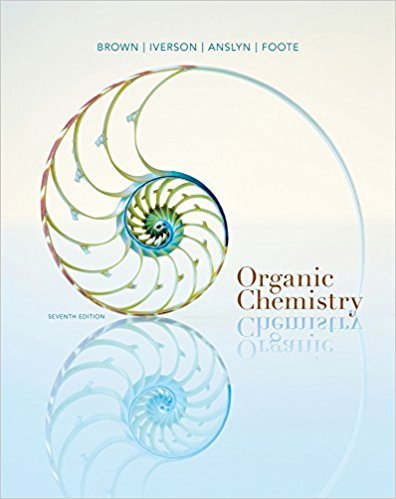×
Get Full Access to Organic Chemistry - 7 Edition - Chapter 2 - Problem 2.29
Get Full Access to Organic Chemistry - 7 Edition - Chapter 2 - Problem 2.29

×

# For each IUPAC name, draw the corresponding structural formula and line-angleISBN: 9781133952848 483

## Solution for problem 2.29 Chapter 2

Organic Chemistry | 7th Edition

• Textbook Solutions
• 2901 Step-by-step solutions solved by professors and subject experts
• Get 24/7 help from StudySoup virtual teaching assistantsOrganic Chemistry | 7th Edition

4 5 1 398 Reviews
17
3
Problem 2.29

For each IUPAC name, draw the corresponding structural formula and line-angle formula.(a) Ethanol (b) Butanal (c) Butanoic acid(d) Ethanoic acid (e) Heptanoic acid (f) Propanoic acid(g) Octanal (h) Cyclopentene (i) Cyclopentanol(j) Cyclopentanone (k) Cyclohexanol (l) Propanone

Step-by-Step Solution:
Step 1 of 3

Week 10 CHEM 1500 10/25 & 10/27 We are 3/5 the way finished with semester! On Tuesday, we learned yet another way to convert to moles. All this section is about is converting to moles. If you get stuck, CONVERT TO MOLES  On Thursday, we took our second exam. I’m sure you all did fine. Enjoy your weekend and don’t let this stress you out. It’s Halloween! HAPPY HALLOWEEN 

Step 2 of 3

Step 3 of 3

##### ISBN: 9781133952848

This textbook survival guide was created for the textbook: Organic Chemistry, edition: 7. The answer to “For each IUPAC name, draw the corresponding structural formula and line-angle formula.(a) Ethanol (b) Butanal (c) Butanoic acid(d) Ethanoic acid (e) Heptanoic acid (f) Propanoic acid(g) Octanal (h) Cyclopentene (i) Cyclopentanol(j) Cyclopentanone (k) Cyclohexanol (l) Propanone” is broken down into a number of easy to follow steps, and 36 words. Organic Chemistry was written by and is associated to the ISBN: 9781133952848. Since the solution to 2.29 from 2 chapter was answered, more than 271 students have viewed the full step-by-step answer. This full solution covers the following key subjects: . This expansive textbook survival guide covers 24 chapters, and 1131 solutions. The full step-by-step solution to problem: 2.29 from chapter: 2 was answered by , our top Chemistry solution expert on 03/16/18, 05:02PM.

## Discover and learn what students are asking

Calculus: Early Transcendental Functions : Conics, Parametric Equations, and Polar Coordinates
?In Exercises 1–6, match the equation with the correct graph. [The graphs are labeled (a), (b), (c), (d), (e), and (f).]

Calculus: Early Transcendental Functions : Functions of Several Variables
?In Exercises 15 - 22, find all first partial derivatives. $$f(x, y)=e^{x} \cos y$$

Unlock Textbook Solution